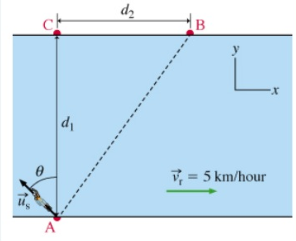# Problem: A swimmer wants to cross a river, from point A to point B, as shown in the figure. The distance (from A to C) is 200 m, the distance (from C to B) is 150 m , and the speed of the current in the river is 5 km/hr . Suppose that the swimmer's velocity relative to the water makes an angle of θ = 45° with the line from A to C, as indicated in the figure.To swim directly from A to B, what speed, relative to the water, should the swimmer have?(Express the swimmer's speed numerically, to three significant figures, in kilometers per hour)As for the figure, it is a right triangle consisting of points A, B, and C. C to B lie on the x-axis and the distance from C to B is labeled "d2". A to C lies on the y-axis and the distance from A to C (point zero) is "d1". Angle θ = 45° and lies at point A.

###### FREE Expert Solution

Let the angle between AB and AC be Φ.

tan Φ = 150/200 = 3/4

The velocity of the water is (5,0)

The y-component of  net velocity is:

vy = uscosθ

The x-component of net velocity is:

vx = 5 - ussinθ

79% (494 ratings)###### Problem Details
A swimmer wants to cross a river, from point A to point B, as shown in the figure. The distance (from A to C) is 200 m, the distance (from C to B) is 150 m , and the speed of the current in the river is 5 km/hr . Suppose that the swimmer's velocity relative to the water makes an angle of θ = 45° with the line from A to C, as indicated in the figure.
To swim directly from A to B, what speed, relative to the water, should the swimmer have?
(Express the swimmer's speed numerically, to three significant figures, in kilometers per hour)
As for the figure, it is a right triangle consisting of points A, B, and C. C to B lie on the x-axis and the distance from C to B is labeled "d2". A to C lies on the y-axis and the distance from A to C (point zero) is "d1". Angle θ = 45° and lies at point A.